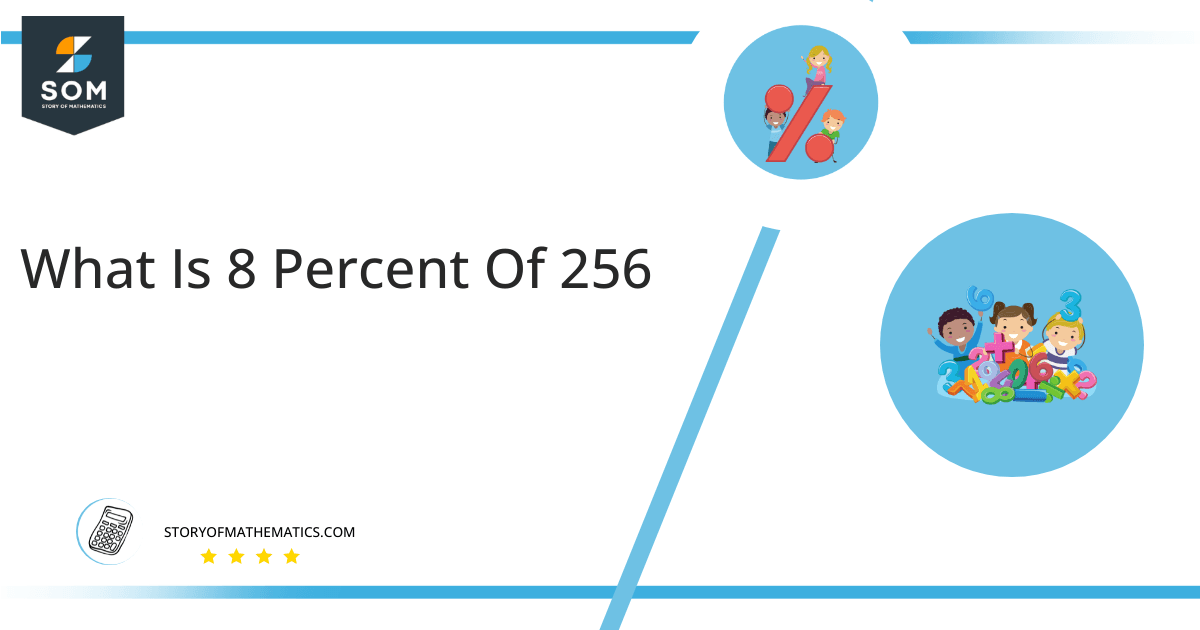# What Is 8 Percent of 256 + Solution with Free Steps?The 8 percent of 256 is equal to 20.48. It can be easily calculated by dividing 8 by 100 and multiplying the answer with 256 to get 20.48.

The easiest way to get this answer is by solving a simple mathematical problem of percentage. You need to find 8% of 256 for some sale or real-life problem. Divide 8 by 100, multiply the answer with 256, and get the 8% of 256 value in seconds.

This article will explain the full process of finding any percentage value from any given quantity or number with easy and simple steps.

## What Is 8 percent of 256?

The 8 percent of 256 is 20.48.

The percentage can be understood with a simple explanation. Take 256, and divide it into 100 equal parts. The 8 number of parts from the total 100 parts is called 8 percent, which is 20.48 in this example.

## How To Calculate 8 percent of 256?

You can find 8 percent of 256 by some simple mathematical steps explained below.### Step 1

Firstly, depict 8 percent of 256 as a fractional multiple as shown below:

8% x 256

### Step 2

The percentage sign % means percent, equivalent to the fraction of 1/100.

Substituting this value in the above formula:

= (8/100) x 256

### Step 3

Using the algebraic simplification process, we can arithmetically manipulate the above equation as follows:

= (8 x 256) / 100

= 2048 / 100

= 20.48This percentage can be represented on a pie chart for visualization. Let us suppose that the whole pie chart represents the 256 value. Now, we find 8 percent of 256, which is 20.48. The area occupied by the 20.48 value will represent the 8 percent of the total 256 value. The remaining region of the pie chart will represent 92 percent of the total 256 value. The 100% of 256 will cover the whole pie chart as 256 is the total value.

Any given number or quantity can be represented in percentages to better understand the total quantity. The percentage can be considered a quantity that divides any number into hundred equal parts for better representation of large numbers and understanding.

Percentage scaling or normalization is a very simple and convenient method of representing numbers in relative terms. Such notations find wide application in many industrial sectors where the relative proportions are used.